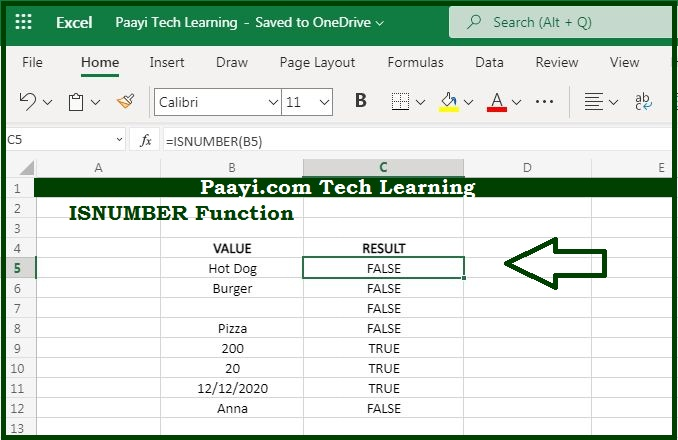# Learn How to Use Microsoft Excel ISNUMBER Function

Written by | 0 Comments | 674 Views

In this article, you will learn how to use the Microsoft Excel ISNUMBER function and its prime function in Microsoft Excel. You will also get to know the Microsoft Excel ISNUMBER function return value and syntax with the help of some examples.

Microsoft Excel ISNUMBER Function

The main function of the Microsoft Excel ISNUMBER function is to test for a numeric value. That means with the help of the ISNUMBER function you can able to get the return value as TRUE if the cell contains a number, and FALSE if there is not any number in the cell. The ISNUMBER function can also be used to check that the cell contains a numeric value, or the result of another function is the number.

Return Value of ISNUMBER Function

The return value will be logical TRUE or FALSE.

Syntax of ISNUMBER Function

=ISNUMBER(value)

Where the arguments:

• value: It is the value to check.

## How to Use Microsoft Excel ISNUMBER Function?The main function of the Microsoft Excel ISNUMBER function is to test for a numeric value. That means with the help of the ISNUMBER function you can able to get the return value as the TRUE if the cell contains a number, and FALSE if there is not any number in the cell. The ISNUMBER function can also be used to check that the cell contains a numeric value, or the result of another function is the number.

It should be noted that:

• Usually, the value is supplied ad a cell address, but you can also wrap other functions and formulas in the ISNUMBER function to test the result.
• The ISNUMBER function will return TRUE for dates and times since they are numeric, and FALSE for numbers is entered as text.
• The ISNUMBER function is the part of the "IS" function.